All You Need to Know About

# Date and Time in Excel

We have a lot of tutorials for you about Excel Date Formulas and Excel Time Formulas. Master them all here!

Here are the top things on what you can do with Excel Date Functions and Excel Time Functions:

## WEEKDAY Formula in Excel

What does it do?

Returns the day of the week corresponding to a date.  The day is given as an integer, ranging from 1 (Sunday) to 7 (Saturday), by default.

Formula breakdown:

=WEEKDAY(Serial_Number, [Return_Type])

What it means:

=WEEKDAY(Date, [Numbers 1 (Sunday) through 7 (Saturday)])

The WEEKDAY function returns the day of the week corresponding to a date.  The day is given as an integer, ranging from 1 (Sunday) to 7 (Saturday).

So if you want to find out on what day you were born, then the WEEKDAY function will remind you.

STEP 1: Enter any date that you want.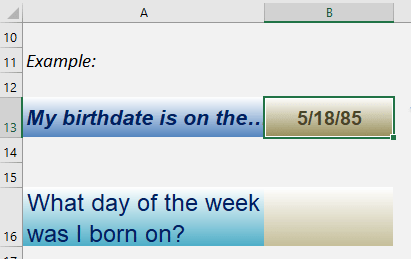STEP 2: The WEEKDAY arguments:

## Date

What is the date?

Select the date you have entered

## =WEEKDAY(B13,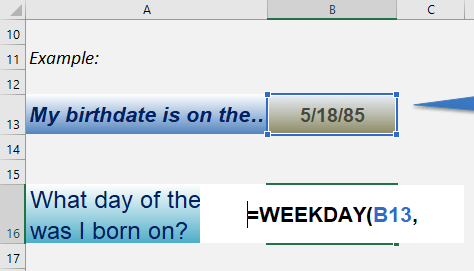## Return_Type

What kind of output?

Type in 1 to get the number of the day of the week

We get a result of 7 here, which signifies a Saturday. As Type 1 represents days ranging from 1 (Sunday) to 7 (Saturday).

## =WEEKDAY(B13, 1)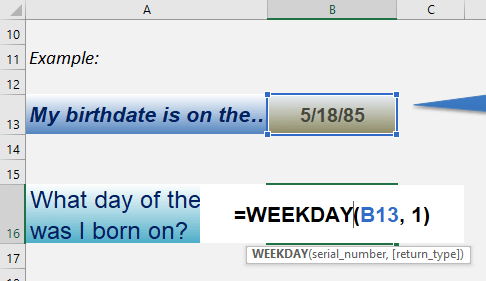Now we know the day in an instant!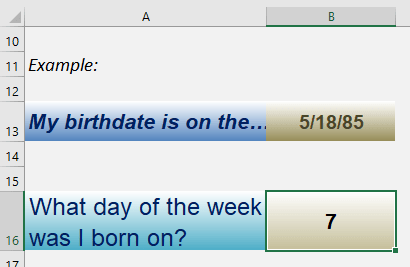## WEEKNUM Formula in Excel

What does it do?

Gets the week number from the date

Formula breakdown:

=WEEKNUM(serial_number, [return_type])

What it means:

=WEEKNUM(date wherein the week number will be retrieved, [day when the week will begin])

Do you need to retrieve the week number from a given date? The WEEKNUM Formula in Excel is perfect for this!

There are a couple of interesting points to take note of the WEEKNUM Formula:

• The return type parameter specifies which day of the week is used to start a new week number. For our examples, we will be using the default. But there are a number of settings that you can use:
• 1 (default) – Sunday
• 2 – Monday
• 11 – Monday
• 12 – Tuesday
• 13 – Wednesday
• 14 – Thursday
• 15 – Friday
• 16 – Saturday
• 17 – Sunday
• 21 – Monday, the difference here is it uses the European week numbering system, week 1 is the week containing the first Thursday of the year as specified in ISO 8601

I explain how you can do this below:

STEP 1: We need to enter the WEEKNUM function in a blank cell:

## =WEEKNUM(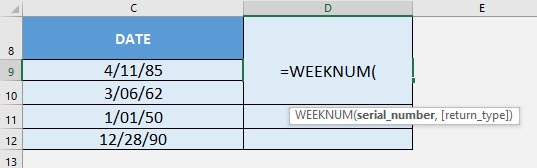STEP 2: The WEEKNUM arguments:

## serial_number

What is the date to extract the week number from?

Select the cell containing the date:

## =WEEKNUM(C9)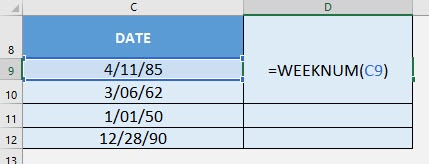Apply the same formula to the rest of the cells by dragging the lower right corner downwards.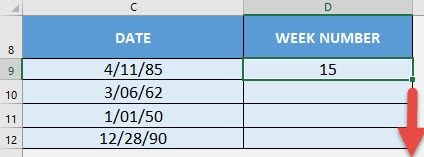You now have your week numbers!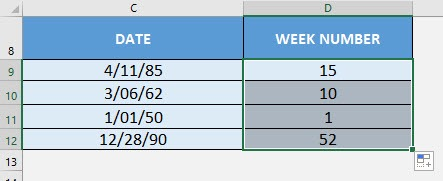## WORKDAY Formula in Excel

What does it do?

Adds/Subtracts a specified number of workdays to a Date, which will give you a Future/Past Date

Formula breakdown:

=WORKDAY(start_date, days, [holidays])

What it means:

=WORKDAY(specified date, number of work days to add/subtract, [holidays to be considered])

If you want to calculate the future date based on a number of working days added, it will be difficult to manually calculate this!

The hard way: I would normally grab my calendar, and count the days one by one!

The easy way: Excel’s WORKDAY formula!

I explain how you can do this below:

STEP 1: We need to enter the WORKDAY function in a blank cell:

## =WORKDAY(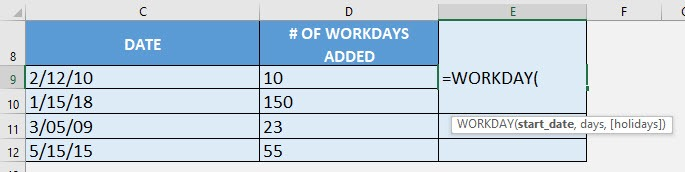STEP 2: The WORKDAY arguments:

## start_date

What is the specified date?

Select the cell containing the date you want to add the number of workdays to:

## =WORKDAY(C9,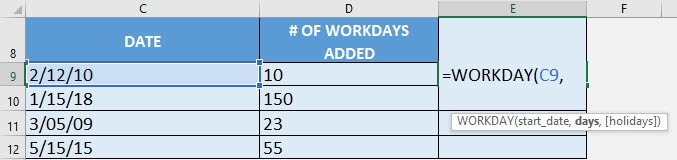## days

How many work days to be added?

Select the cell containing the number of work days to be added (i.e The number of non-weekend and non-holiday days after the start date):

## =WORKDAY(C9, D9)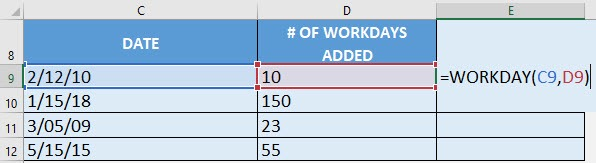Apply the same formula to the rest of the cells by dragging the lower right corner downwards.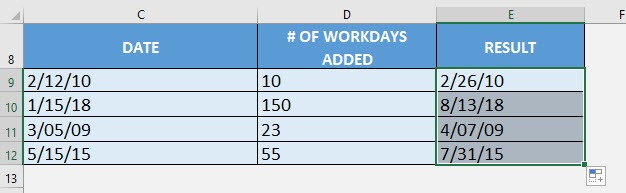Latest Tutorials

March 3, 2021

[l]
[l]
[l]
[l]
[l]
[l]
[l]
[l]
[l]
[l]
[l]
[l]
[l]
[l]
[l]
[l]
[l]
[l]
[l]
[l]
[l]
[l]
[l]
[l]
[l]
[l]
[l]
[l]
[l]
[l]